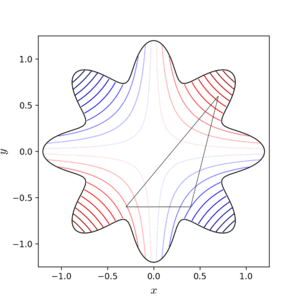# Mathematical optimization facts for kids

Kids Encyclopedia Facts
(Redirected from Optimization (mathematics))Nelder-Mead minimum search of Simionescu's function. Simplex vertices are ordered by their value, with 1 having the lowest (best) value.

In many branches of science, including mathematics, mathematical optimization is a branch that is about finding the element that gives an optimal solution to a problem, given some criteria. In the simplest case, this means that a function needs to be minimized or maximized.

Many problems are more complex, though, and solving them analytically is not feasible: in these cases, numerical methods are often used.Mathematical optimization Facts for Kids. Kiddle Encyclopedia.